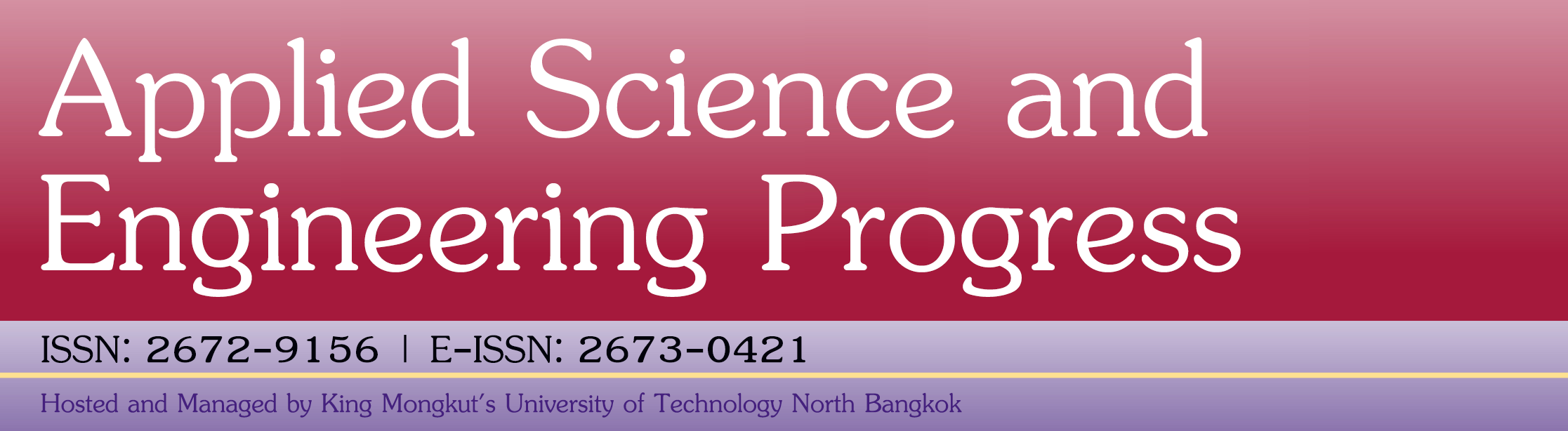Model-robust G-optimal Designs in the Presence of Block Effects

Peang-or Yeesa, Patchanok Srisuradetchai, John J. Borkowski

Abstract

Due to the uncertainty of possible reduced models prior to data collection, this paper considered using experimental designs that are robust across the set of potential models. In this study, blocking effects were combined into all possible models which were obtained from weak heredity principle (WH). The objective of this article was to propose the geometric mean of G-optimalities as a new alternative for finding robust response surface designs against model misspecification. The proposed criterion is so-called a weighted G-optimality criterion (Gw). The genetic algorithm (GA) was employed to optimize the weighted G-optimality criterion for finding designs. Robust designs having 2 and 3 design variables in hypercube were generated with an appropriate number of design points in each blocks and the number of blocks in this study are 2, 3, and 4. The scheme for weighting the criteria was to give more weight to a model with a larger number of parameters. The resulting weighted G-optimal designs have higher G-efficiencies compared to those of G-optimal designs if a true model is the first-order or interaction models. The G-efficiency of a weighted G-optimal design is slightly less than that of a G-optimal design even when the true model is a second-order model. Furthermore, design points identified from the GA are also presented, which would be very useful in practice for those intending to implement an experimental design for data collection.

Keywords

Full Text: PDF

DOI: 10.14416/j.asep.2019.07.001